## B.2 Multi-path fading Propagation Conditions

36.521-13GPPEvolved Universal Terrestrial Radio Access (E-UTRA)Part 1: Conformance testingRadio transmission and receptionRelease 17TSUser Equipment (UE) conformance specification

The multipath propagation conditions consist of several parts:

– A delay profile in the form of a "tapped delay-line", characterized by a number of taps at fixed positions on a sampling grid. The profile can be further characterized by the r.m.s. delay spread and the maximum delay spanned by the taps.

– A combination of channel model parameters that include the Delay profile and the Doppler spectrum that is characterized by a classical spectrum shape and a maximum Doppler frequency

– A set of correlation matrices defining the correlation between the UE and eNodeB antennas in case of multi-antenna systems.

## B.2.1 Delay profiles

The delay profiles are selected to be representative of low, medium and high delay spread environments. The resulting model parameters are defined in Table B.2.1-1 and the tapped delay line models are defined in Tables B.2.1-2, B.2.1-3 and B.2.1-4.

Table B.2.1-1: Delay profiles for E-UTRA channel models

 Model Number of channel taps Delay spread (r.m.s.) Maximum excess tap delay (span) Extended Pedestrian A (EPA) 7 45 ns 410 ns Extended Vehicular A model (EVA) 9 357 ns 2510 ns Extended Typical Urban model (ETU) 9 991 ns 5000 ns

Table B.2.1-2: Extended Pedestrian A model (EPA)

 Excess tap delay [ns] Relative power [dB] 0 0.0 30 -1.0 70 -2.0 90 -3.0 110 -8.0 190 -17.2 410 -20.8

Table B.2.1-3: Extended Vehicular A model (EVA)

 Excess tap delay [ns] Relative power [dB] 0 0.0 30 -1.5 150 -1.4 310 -3.6 370 -0.6 710 -9.1 1090 -7.0 1730 -12.0 2510 -16.9

Table B.2.1-4: Extended Typical Urban model (ETU)

 Excess tap delay [ns] Relative power [dB] 0 -1.0 50 -1.0 120 -1.0 200 0.0 230 0.0 500 0.0 1600 -3.0 2300 -5.0 5000 -7.0

## B.2.2 Combinations of channel model parameters

The propagation conditions used for the performance measurements in multi-path fading environment are indicated as EVA[number], EPA[number] or ETU[number] where ‘number’ indicates the maximum Doppler frequency (Hz).

Table B.2.2-1: Void

## B.2.3 MIMO Channel Correlation Matrices

The MIMO channel correlation matrices defined in B.2.3 apply for the antenna configuration using uniform linear arrays at both eNodeB and UE.

### B.2.3.1 Definition of MIMO Correlation Matrices

Table B.2.3.1-1 defines the correlation matrix for the eNodeB

Table B.2.3.1-1: eNodeB correlation matrix

 One antenna Two antennas Four antennas eNode B Correlation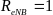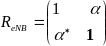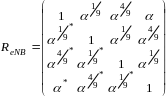Table B.2.3.1-2 defines the correlation matrix for the UE:

Table B.2.3.1-2: UE correlation matrix

 One antenna Two antennas Four antennas UE Correlation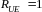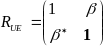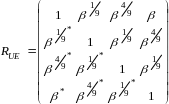Table B.2.3.1-3 defines the channel spatial correlation matrix . The parameters, α and β in Table B.2.3.1-3 defines the spatial correlation between the antennas at the eNodeB and UE.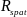Table B.2.3.1-3: correlation matrices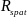1×2 case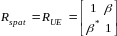2×1 case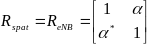2×2 case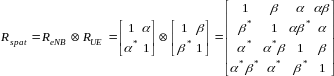4×2 case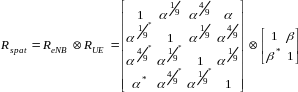4×4 case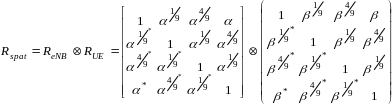For cases with more antennas at either eNodeB or UE or both, the channel spatial correlation matrix can still be expressed as the Kronecker product of and according to.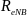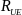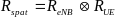### B.2.3.2 MIMO Correlation Matrices at High, Medium and Low Level

The and for different correlation types are given in Table B.2.3.2-1.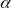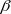Table B.2.3.2-1

 Low correlation Medium Correlation High Correlation α β α β α β 0 0 0.3 0.9 0.9 0.9

The correlation matrices for high, medium and low correlation are defined in Table B.2.3.2-2, B.2.3.2-3 and B.2.3.2-4,as below.

The values in the Table B.2.3.2-2 table have been adjusted for the 4×2 and 4×4 high correlation cases to insure the correlation matrix is positive semi-definite after round-off to 4 digit precision. This is done using the equation: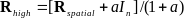Where the value “a” is a scaling factor such that the smallest value is used to obtain a positive semi-definite result. For the 4×2 high correlation case, a=0.00010. For the 4×4 high correlation case, a=0.00012.

The same method is used to adjust the 4×4 medium correlation matrix in Table B.2.3.2-3 to insure the correlation matrix is positive semi-definite after round-off to 4 digit precision with a = 0.00012.

Table B.2.3.2-2: MIMO correlation matrices for high correlation

 1×2 case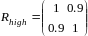2×1 case2×2 case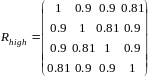4×2 case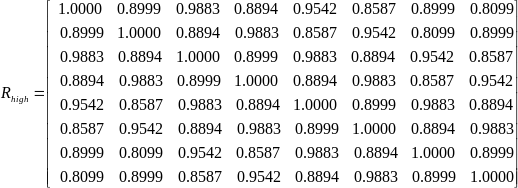4×4 case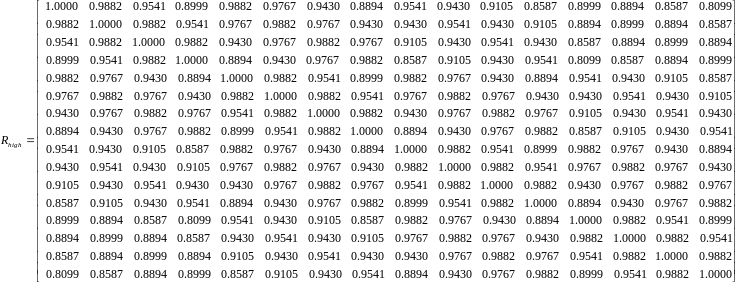Table B.2.3.2-3: MIMO correlation matrices for medium correlation

 1×2 case N/A 2×1 case N/A 2×2 case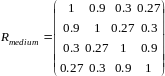4×2 case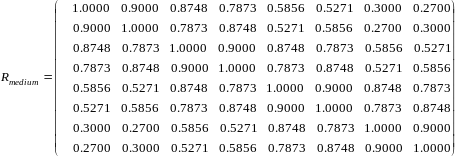4×4 case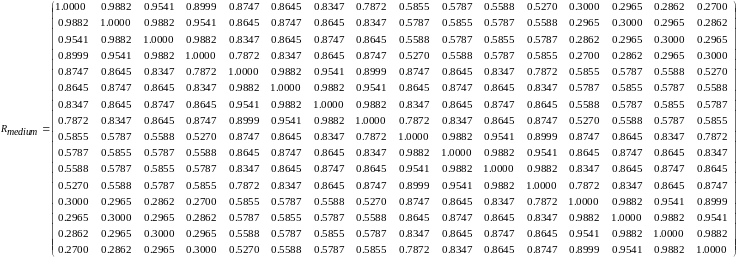Table B.2.3.2-4: MIMO correlation matrices for low correlation

 1×2 case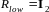2×1 case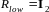2×2 case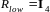4×2 case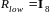4×4 case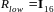In Table B.2.3.2-4,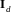is the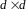identity matrix.

## B.2.3A MIMO Channel Correlation Matrices using cross polarized antennas

The MIMO channel correlation matrices defined in B.2.3A apply for the antenna configuration using cross polarized (XP/X-pol) antennas at both eNodeB and UE. The cross-polarized antenna elements with +/-45 degrees polarization slant angles are deployed at eNB and cross-polarized antenna elements with +90/0 degrees polarization slant angles are deployed at UE.

For the cross-polarized antennas, the N antennas are labelled such that antennas for one polarization are listed from 1 to N/2 and antennas for the other polarization are listed from N/2+1 to N, where N is the number of transmit or receive antennas.

#### B.2.3A.1 Definition of MIMO Correlation Matrices using cross polarized antennas

For the channel spatial correlation matrix, the following is used: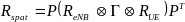Where

– is the spatial correlation matrix at the UE with same polarization,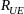– is the spatial correlation matrix at the eNB with same polarization,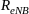– is a polarization correlation matrix, and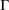– denotes transpose.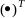The matrix is defined as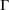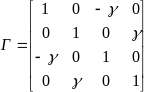A permutation matrix element is defined as: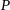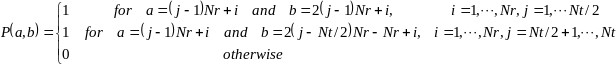Where Nt and Nr is the number of transmitter and receiver respectively. This is used to map the spatial correlation coefficients in accordance with the antenna element labelling system described in B.2.3A.

#### B.2.3A.2 Spatial Correlation Matrices using cross polarized antennas at eNB and UE sides

##### B.2.3A.2.1 Spatial Correlation Matrices at eNB side

For 2-antenna transmitter using one pair of cross-polarized antenna elements,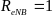.

For 4-antenna transmitter using two pairs of cross-polarized antenna elements,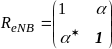.

For 8-antenna transmitter using four pairs of cross-polarized antenna elements,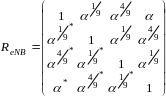.

##### B.2.3A.2.2 Spatial Correlation Matrices at UE side

For 2-antenna transmitter using one pair of cross-polarized antenna elements,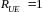.

For 4-antenna transmitter using two pairs of cross-polarized antenna elements,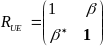.

#### B.2.3A.3 MIMO Correlation Matrices using cross polarized antennas

The values for parameters α, β and γ for low correlation and high spatial correlation are given in Table B.2.3A.3-1.

Table B.2.3A.3-1: The and parameters for cross-polarized MIMO correlation matrices

 Correlation Model α β  Medium Correlation A 0.3 0.6 0.2 High Correlation 0.9 0.9 0.3 Note 1: Value of α applies when more than one pair of cross-polarized antenna elements at eNB side. Note 2: Value of β applies when more than one pair of cross-polarized antenna elements at UE side.

The correlation matrices for high spatial and low correlation are defined in Table B.2.3A.3-2 and Table B.2.3A.3-3 as below.

The values in Table B.2.3A.3-2 have been adjusted to insure the correlation matrix is positive semi-definite after round-off to 4 digit precision. This is done using the equation: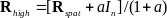Where the value "a" is a scaling factor such that the smallest value is used to obtain a positive semi-definite result. For the 8×2 high spatial correlation case, a=0.00010.

Table B.2.3A.3-2: MIMO correlation matrices for high spatial correlation

 4×2 case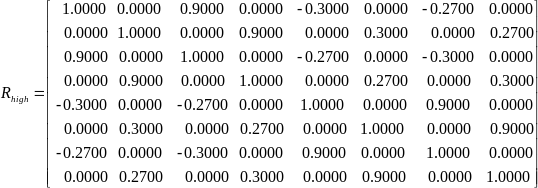8×2 case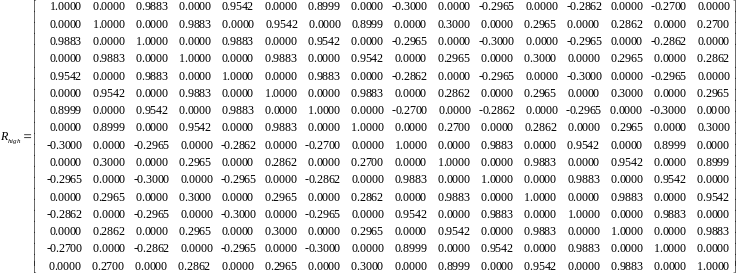Table B.2.3A.3-3: MIMO correlation matrices for medium correlation A

 4×4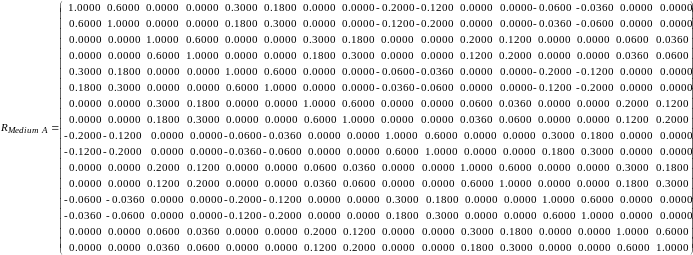#### B.2.3A.4 Beam steering approach

Given the channel spatial correlation matrix in B.2.3A.1, the corresponding random channel matrix H can be calculated. The signal model for the k-th subframe is denoted as: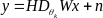Where

– H is the N¬r xNt channel matrix per subcarrier.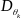is the steering matrix,

For 8 transmission antennas,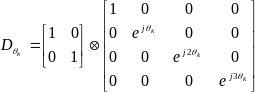;

For 4 transmission antennas,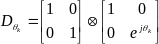.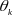controls the phase variation, and the phase for k-th subframe is denoted by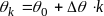, where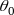is the random start value with the uniform distribution, i.e.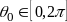,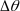is the step of phase variation, which is defined in Table B.2.3A.4-1, and k is the linear increment of 1 for every subframe throughout the simulation,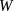is the precoding matrix for Nt transmission antennas,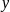is the received signal,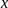is the transmitted signal, and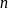is AWGN.

Table B.2.3A.4-1: The step of phase variation

 Variation Step Value (rad/subframe)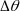1.2566×10-3

## B.2.4 Propagation conditions for CQI tests

[For Channel Quality Indication (CQI) tests, the following additional multi-path profile is used: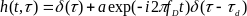in continuous time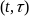representation, with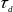the delay, a a constant and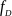the Doppler frequency.]

## B.2.6 MBSFN Propagation Channel Profile

Table B.2.6-1 shows propagation conditions that are used for the MBSFN performance requirements in multi-path fading environment in an extended delay spread environment.

Table B.2.6-1: Propagation Conditions for Multi-Path Fading Environments for MBSFN Performance Requirements in an extended delay spread environment

 Extended Delay Spread Maximum Doppler frequency [5Hz] Relative Delay [ns] Relative Mean Power [dB] 0 0 30 -1.5 150 -1.4 310 -3.6 370 -0.6 1090 -7.0 12490 -10 12520 -11.5 12640 -11.4 12800 -13.6 12860 -10.6 13580 -17.0 27490 -20 27520 -21.5 27640 -21.4 27800 -23.6 27860 -20.6 28580 -27.0